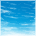## SGTL5000 Sample Rate Master mode Calculation

cancel
Showing results for
Did you mean:

## SGTL5000 Sample Rate Master mode Calculation

617 ViewsContributor I

We want to configure SGTL5000 as master for 8 KHz sample rate with our custom board .

My SGTL5000 version is 0XA011.

VDDIO = VDDA = 3.3 V and externally applied VDDD is around 1.8 V

In our environment,

First Scenario - Asynchronous System Main Clock

SYS_FS = 48 KHz  , RATE_MODE = 0x3 (1/6 of SYS_FS rate)

provided System main clock = 12 MHz,

SCLKFREQ = 32Fs

PLL used with integer divisor and fractional divisor equal to 16 and 786 respectively.

With this configuration , bit-clock value is equal to 512 KHz and Sample Clock(LR clock) is 16 KHz.

As mentioned in the datasheet , sample clock value should be 8 KHz .

Second Scenario - Synchronous System Main Clock

SYS_FS = 48 KHz , RATE_MODE = 0x1 (1/2 of SYS_FS rate)

provided System Main Clock = 2.048 MHz,

SCLKFREQ = 32 Fs

PLL not used

VDDIO = VDDA = 3.3 V and externally applied VDDD is around 1.8 V

With this configuration , bit clock I am getting around 256 KHz and Sample Clock(LR clock) is 8 KHz .

From this Datasheet ,

SYS_FS / RATE_MODE = Fs (Sampling Frequency)

Is there anything I am missing here or else what are the required steps to configure sample clock equal to 8 KHz in master mode ??

- Pinkesh

Tags (1)
4 Replies
158 ViewsNXP TechSupport

1. For PLL mode, the SYS_FS / RATE_MODE = Fs equation is only true with SCLKFREQ setting of 64Fs (SCLKFREQ=0). For SCLKFREQ setting of 32Fs (as in your case), Fs is higher twice. So, for PLL mode and SCLKFREQ of 32Fs, when calculating the PLL settings, use the SYS_MCLK frequency value that is a half of the actual input frequency.

2. In synchronous mode, the the frequency of the SCLK bit clock just directly depends on the frequency of the SYS_MCLK input clock. It can be calculated as follows depending on the MCLK_FREQ bit settings.

SCLK = SYS_MCLK/8    for MCLK_FREQ = 0 (256Fs)

SCLK = SYS_MCLK/12    for MCLK_FREQ = 1 (384Fs)

SCLK = SYS_MCLK/16    for MCLK_FREQ = 2 (512Fs)

Then, the Fs frequency can be calculated as SCLK/32 or SCLK/64 depending on the SCLKFREQ bit setting.

Have a great day,
Artur

-----------------------------------------------------------------------------------------------------------------------
-----------------------------------------------------------------------------------------------------------------------

158 ViewsContributor I

Thanks Arthur for the info .

So, for PLL mode and SCLKFREQ of 32Fs, when calculating the PLL settings, use the SYS_MCLK frequency value that is a half of the actual input frequency .

Arthur I think there is something not clear about input freq.Actually in our program , We take the double the value of actual input frequency which makes integer divisor 8 .

After this , we are getting sample clock as required (8 KHz).

158 ViewsContributor I

Hello Pinkesh,

I also want to configure the SGTL5000 in master mode. Can you please share your SGTL5000 configuration code ?